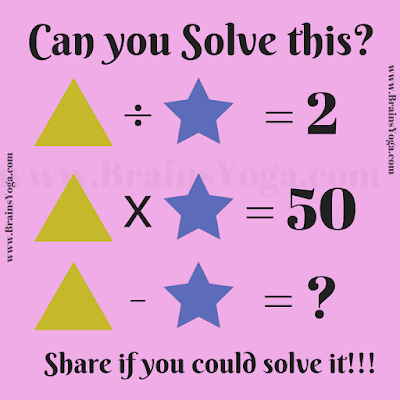Here is a simple Mathematical equation for kids. In this Maths equation, your challenge is to find the value of a given variable Triangle, or Star, and then solve the last equation. Can you solve the Maths Puzzle as quickly as possible?Can you solve this Maths Equation?